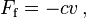# Damping facts for kids

Kids Encyclopedia Facts

In physics, damping is any effect that tends to reduce the amplitude of vibrations.

In mechanics, the internal friction may be one of the causes of such damping effect. For many purposes the damping force Ff can be modeled as being proportional to the velocity v of the object:$F_{\mathrm f} = -c v \, ,$

where c is the damping coefficient, given in units of Newton-seconds per meter.

## Related pagesDamping Facts for Kids. Kiddle Encyclopedia.